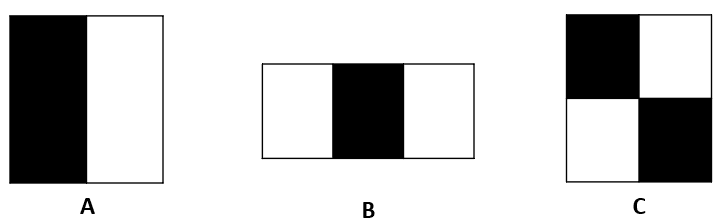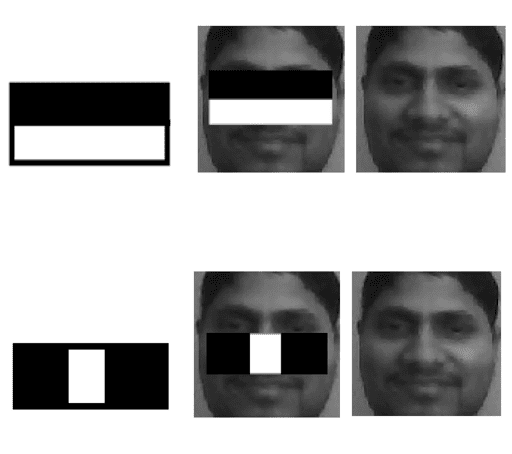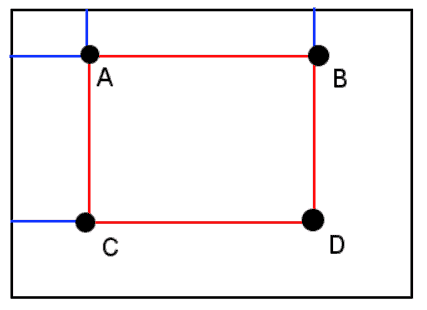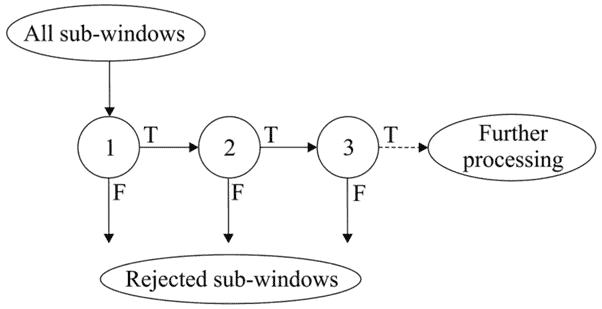## 1. Overview

In this tutorial, we’ll review the Viola-Jones algorithm, a popular machine-learning method for object detection. We’ll provide a simple explanation of the key ideas of the algorithm.

## 2. What Is Viola-Jones Algorithm?

Viola-Jones algorithm is a machine-learning technique for object detection proposed in 2001 by Paul Viola and Michael Jones in their paper “Rapid object detection using a boosted cascade of simple features”. The algorithm was primarily conceived for face detection. Despite it has lower accuracy than modern face detection methods based on Convolutional Neural Networks (CNNs), the Viola-Jones algorithm is still an efficient solution for resource-constrained devices.

Given a grayscale image, the algorithm analyzes many windows of different sizes and positions and tries to detect the target object by looking for specific image features in each window.

The Viola-Jones algorithm is based on four main ideas, which we’ll discuss in the sections below:

• Haar-like features
• Integral images for accelerating the feature computation
• AdaBoost learning for feature selection
• Cascade of classifiers for fast rejection of windows without faces.

## 3. What Are Haar-Like Features?

The Viola-Jones algorithm uses a set of features similar to Haar wavelets, which are a set of square-shaped functions. More specifically, the algorithm uses three types of Haar-like features represented in the following figure:Each feature specifies a set of rectangles in the image window. A rectangle can be marked as white or black. The feature value is computed as the difference between the sum of the pixel values in the white areas and the sum of pixel values in the black areas.

The feature value will be around zero for “flat regions”, i.e., where all the pixels have the same value. A large feature value will be obtained in the regions where the pixels in the black and white rectangles are very different.

As shown below, features A and B have great importance in face detection since:

• the eye region is darker than the cheeks
• the eye region is darker than the nose region## 4. Integral Image

The integral image is a data structure for efficiently computing the sum of pixel values in a rectangular image window. Hence, the Haar-like features can be computed very quickly using the integral image representation.

Given a grayscale image, the integral image valueat the pointis the sum of all the pixels above and to the left of, inclusive:

(1)The integral image can be computed in a single pass over the imagewith the following equation:

(2)Given an image withpixel, the time complexity of the integral image computation is.

It can be demonstrated that the sum in any rectangular area requires four values of the integral image, regardless of the window size:More specifically, the sum of pixel values within the rectangle ABCD shown above can be computed as:

(3)Hence, using this formula, the time complexity of the computation of the Haar-like feature is.

The number of features is approximately 16000 if the base resolution of the detector is 24×24. However, a small number of these features are useful for detecting faces. Viola-Jones algorithm uses AdaBoost to find the best features and to train a classifier. Each Haar-like feature represents a weak classifier. The final classifier is given by a linear combination of weak classifiers. Larger weights are associated with better classifiers using the AdaBoost learning algorithm.

The attentional cascade is another trick of the Viola-Jones algorithm that allows for an increase in the speed of the detection.

The best feature selected by AdaBoost rejects many negative windows while detecting almost all positive windows. Hence the classifier corresponding to the best feature is evaluated first on a given window. A positive response triggers the evaluation of a second (more complex) classifier, and so on. A negative response at any level leads to the rejection of the window.

This strategy rejects as many negative windows as possible at the earliest stage. Only positive instances trigger all classifiers in the cascade.

The following figure represents the detection cascade schematically:## 7. Conclusion

In this tutorial, we reviewed the Viola-Jones algorithm, an efficient machine-learning algorithm for object detection. We have explained the four key ideas on which the technique is based.